# p范数空间中的旋转几何与物理运动

//注：本文于2020年8月大幅改动并加入了大量新内容！
//注：本文于2023年1月修正了最后一小节关于引力轨道计算的错误并做了相应补充！

## 不一样的距离公式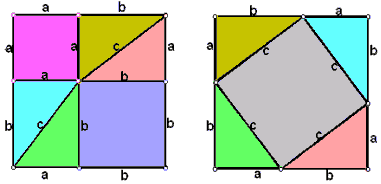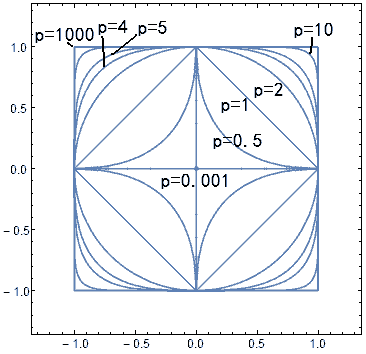$$\begin{pmatrix} x’ \\ y’ \end{pmatrix} = \begin{pmatrix} cos\theta & sin\theta \\ -sin\theta & cos\theta \end{pmatrix} \begin{pmatrix} x \\ y \end{pmatrix} =\begin{pmatrix} x cos\theta+y sin\theta \\ -x sin\theta+y cos\theta \end{pmatrix}$$

## 闵可夫斯基空间

$$\begin{pmatrix} x’ \\ t’ \end{pmatrix} = \begin{pmatrix} cosh\theta & -sinh\theta \\ -sinh\theta & cosh\theta \end{pmatrix} \begin{pmatrix} x \\ t \end{pmatrix}$$

## 广义三角函数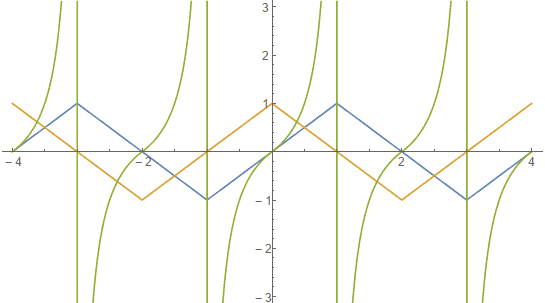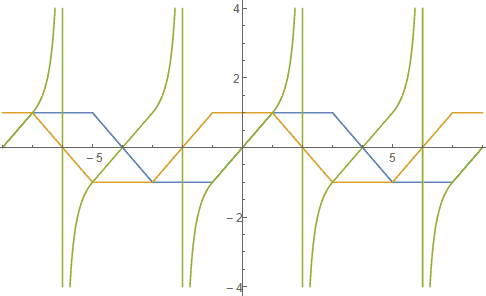1. 最保险是使用弧长的定义，经繁琐计算我们能得到这样的微分方程组：（注意我们的微分方程中必须加入符号函数来谨慎处理距离的定义中的绝对值符号）
$${d sin_p x\over d x}=sign(cos_p x)(1+|sin_p x/cos_p x|^{p(p-1)})^{1-p\over p}$$$${d cos_p x\over d x}=-sign(sin_p x)(1+|cos_p x/sin_p x|^{p(p-1)})^{1-p\over p} x$$$$sin_p(0)=0、cos_p(0)=1$$

2. 根据面积的定义，我们能够算出另一个微分方程组：$${d sin_p x\over d x}=sign((1-sin_p^p x)^{1-p\over p})|(1-sin_p^p x)^{1-p\over p}|=sign(cos_p x)|cos_p^{p-1} x|$$$${d cos_p x\over d x}=-sign(1-cos_p^p x)|(1-cos_p^p x)^{1-p\over p}|=-sign(sin_p x)|sin_p^{p-1} x |$$$$sin_p(0)=0、cos_p(0)=1$$

## [点击展开/收起]广义三角函数的解析性质

### 不同范数下的圆周率与三角函数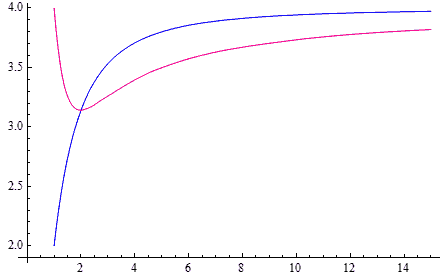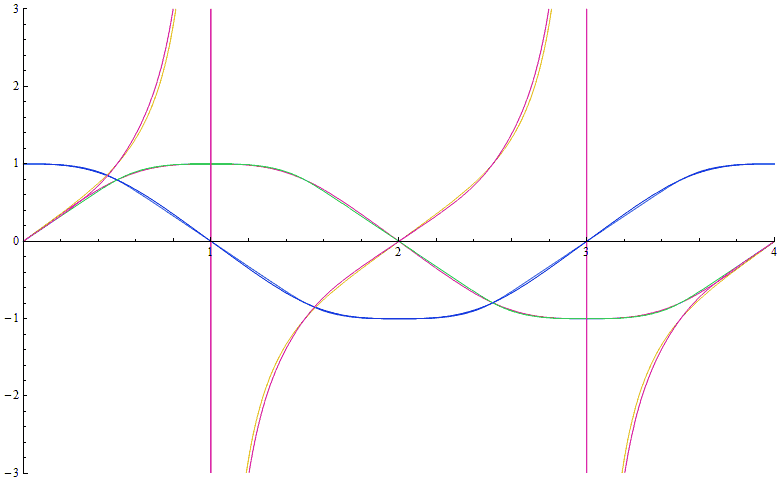## 旋转对称性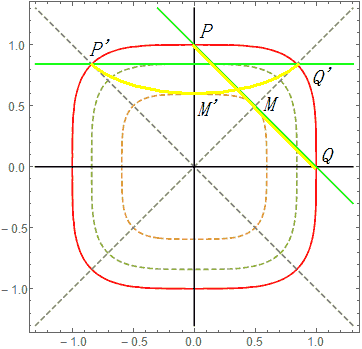$$r=\sqrt[p]{|x|^p+|y|^p}$$$$\theta=atan2_p(y,x)$$$$x’=r cos_p(\theta+\alpha)$$$$y’=r sin_p(\theta+\alpha)$$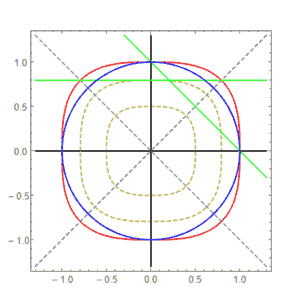## p-范数世界中的弹簧

$$F = -k\Delta x$$其中$\Delta x$是弹簧的伸长量，既然涉及到长度，我们自然就要用上p-范数的距离公式来计算，这样就得到了p-范数世界中的弹簧模型。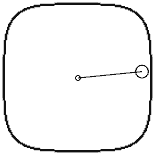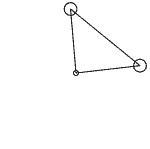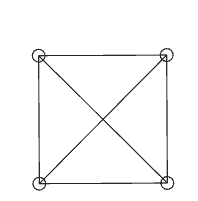## p-范数世界中的天体运动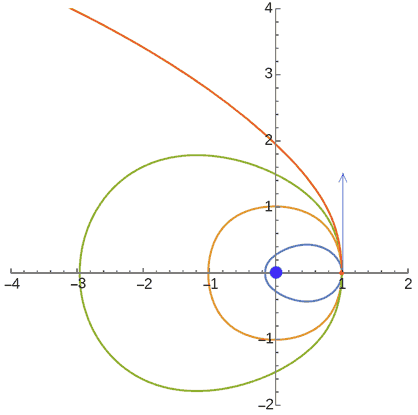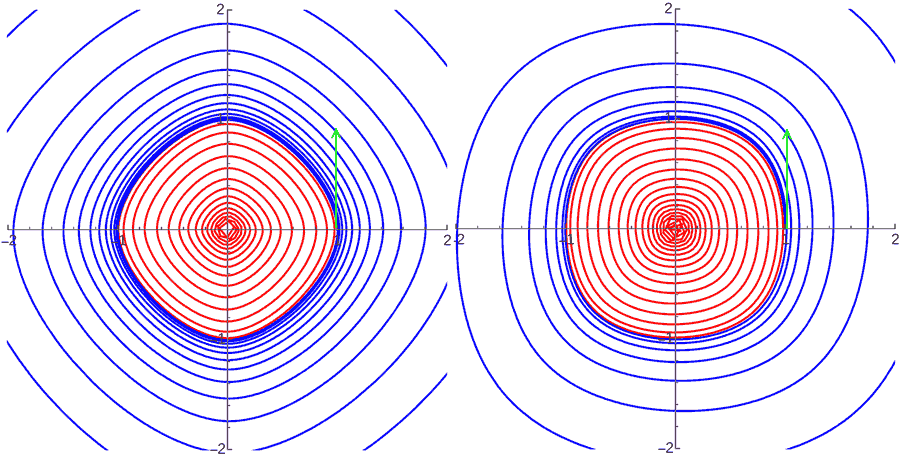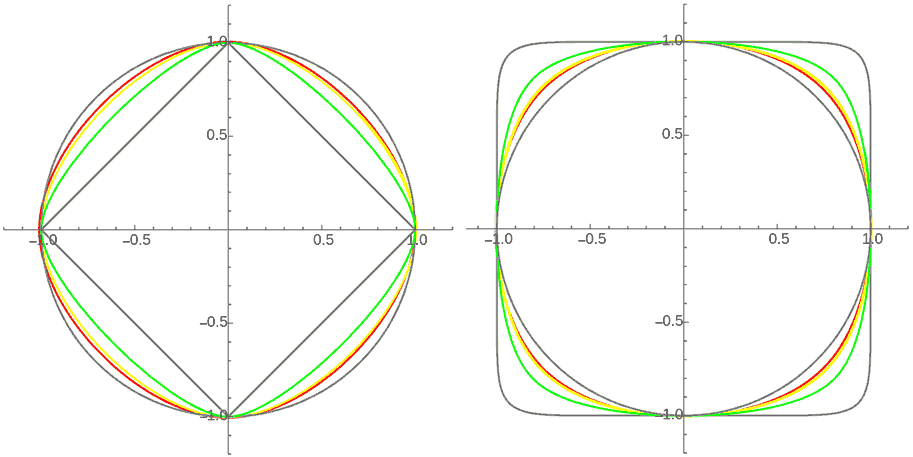## 其它怪异几何

Finsler几何是把切空间上的内积换成了一般的范数，每个切空间不再是一个内积空间，而仅仅是一个赋范线性空间。